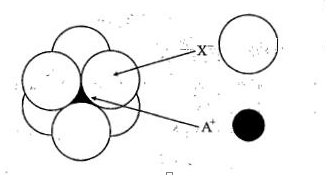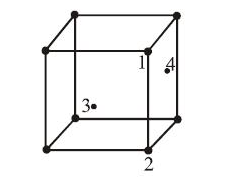If NaCl is doped with 10-4 mol % of SrCl2, the concentration of cation vacancies will be (NA = 6.023 x 1023 mol-1)

(a) 6.023 x 1015 mol-1              (b) 6.023 x 1016 mol-1

(c) 6.023 x 1017 mol-1              (d) 6.023 x 1014 mol-1

Concept Questions :-

Density/Formula/packing fraction/ Semiconductors
High Yielding Test Series + Question Bank - NEET 2020

Difficulty Level:

CsCl crystallises in body-centred cubic lattice. If 'a' is its edge length then which of the following expressions is correct?

(a) rCs+ + rCl- = 3a

(b)  rCs+ + rCl- = 3a/2

(c)  rCs+ + rCl- = ($\sqrt{3}$/2)*a

(d) rCs+ + rCl-$\sqrt{3a}$

Concept Questions :-

Introduction and Type of Crystal System
High Yielding Test Series + Question Bank - NEET 2020

Difficulty Level:

The phenomenon in which polar crystals on heating produce electricity is called:-
1. pyro-electricity
2. piezo-electricity
3. ferro-electricity
4. ferri-electricity

Concept Questions :-

Magnetic Behaviour of an Element/Compound
High Yielding Test Series + Question Bank - NEET 2020

Difficulty Level:

Metals have conductivity of the order of (ohm-1 cm-1):-

(a)1012
(b)108
(c)102
(d)10-6

Concept Questions :-

Density/Formula/packing fraction/ Semiconductors
High Yielding Test Series + Question Bank - NEET 2020

Difficulty Level:

Total volume of atoms present in a face centred cubic unit cell of a metal is ( r is atomic radius):

(A) 20/3 $\pi$r3

(B)  24/3 $\pi$r3

(C)  12/3 $\pi$r3

(D)  16/3 $\pi$r3

Concept Questions :-

High Yielding Test Series + Question Bank - NEET 2020

Difficulty Level:

Lithium borohydride (LiBH4), crystallises in an orthorhombic system with 4 molecules per unit cell. The unit cell dimensions are:- a = 6.81 Å , b = 4.43 Å ,c = 7.17 Å. If the molar mass of LiBH4 is
21.76 g mol-1.The density of the crystal is:-

1. 0.668 gcm-3
2. 0.585 gcm2
3. 1.23 gcm-3

4. None of these

Concept Questions :-

Density/Formula/packing fraction/ Semiconductors
High Yielding Test Series + Question Bank - NEET 2020

Difficulty Level:

The arrangement of Xions around A+ ion in solid AX is given in the figure (not drawn to scale).
If the radius of X is 250 pm,the radius of A+ is:(a)104 pm
(b)125 pm
(c)183 pm
(d) 57 pm

Concept Questions :-

High Yielding Test Series + Question Bank - NEET 2020

Difficulty Level:

In an f.c.c. unit cell. atoms are numbered as shown below.The atoms not touching each other are (Atom numbered 3 is face centre of front face).(A) 3&4
(B) 1&3
(C) 1&2
(D) 2&4

Concept Questions :-

Introduction and Type of Crystal System
High Yielding Test Series + Question Bank - NEET 2020

Difficulty Level:

A solid has a bcc structure. If the distance of closest approach between the two atoms is 1.73Å . The edge length of the cell is:

(a) 200pm

(b) $\sqrt{3}}{\sqrt{2}}$ pm

(c) 142.2 pm

(d) $\sqrt{2}$pm

Concept Questions :-

Density/Formula/packing fraction/ Semiconductors
High Yielding Test Series + Question Bank - NEET 2020

Difficulty Level:

Which is covalent solid?

(a) Fe2O3

(b) Diamond

(c) Graphite

(d) All of these

Concept Questions :-

Introduction and Type of Crystal System